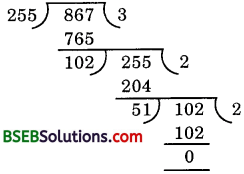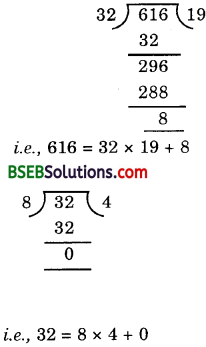# HSSlive: Plus One & Plus Two Notes & Solutions for Kerala State Board

## BSEB Class 10 Maths Chapter 1 Real Numbers Ex 1.1 Textbook Solutions PDF: Download Bihar Board STD 10th Maths Chapter 1 Real Numbers Ex 1.1 Book AnswersBSEB Class 10 Maths Chapter 1 Real Numbers Ex 1.1 Textbook Solutions PDF: Download Bihar Board STD 10th Maths Chapter 1 Real Numbers Ex 1.1 Book Answers

BSEB Class 10th Maths Chapter 1 Real Numbers Ex 1.1 Textbooks Solutions and answers for students are now available in pdf format. Bihar Board Class 10th Maths Chapter 1 Real Numbers Ex 1.1 Book answers and solutions are one of the most important study materials for any student. The Bihar Board Class 10th Maths Chapter 1 Real Numbers Ex 1.1 books are published by the Bihar Board Publishers. These Bihar Board Class 10th Maths Chapter 1 Real Numbers Ex 1.1 textbooks are prepared by a group of expert faculty members. Students can download these BSEB STD 10th Maths Chapter 1 Real Numbers Ex 1.1 book solutions pdf online from this page.

## Bihar Board Class 10th Maths Chapter 1 Real Numbers Ex 1.1 Books Solutions

 Board BSEB Materials Textbook Solutions/Guide Format DOC/PDF Class 10th Subject Maths Chapter 1 Real Numbers Ex 1.1 Chapters All Provider Hsslive

## How to download Bihar Board Class 10th Maths Chapter 1 Real Numbers Ex 1.1 Textbook Solutions Answers PDF Online?

2. Click on the Bihar Board Class 10th Maths Chapter 1 Real Numbers Ex 1.1 Answers.
3. Look for your Bihar Board STD 10th Maths Chapter 1 Real Numbers Ex 1.1 Textbooks PDF.
4. Now download or read the Bihar Board Class 10th Maths Chapter 1 Real Numbers Ex 1.1 Textbook Solutions for PDF Free.

## BSEB Class 10th Maths Chapter 1 Real Numbers Ex 1.1 Textbooks Solutions with Answer PDF Download

Find below the list of all BSEB Class 10th Maths Chapter 1 Real Numbers Ex 1.1 Textbook Solutions for PDF’s for you to download and prepare for the upcoming exams:

## BSEB Bihar Board Class 10th Maths Solutions Chapter 1 Real Numbers Ex 1.1

Question 1.
Use Euclid’s division algorithm to find the HCF of:

(i) 135 and 225
(ii) 196 and 38220
(iii) 867 and 255

Solution:

(i) We have:
Dividend = 225 and Divisor = 135
The process can be exhibited as under:Hence, HCF (135, 225) = 45.

(ii) We have:
Dividend = 38220 and Divisor = 196
38220 = 196 × 195 + 0
Hence, HCF (196, 38220) = 196.(iii) We have:
Dividend = 867 and Divisor = 255Hence, HCF (255, 867) = 51.

Question 2.
Show that any positive odd integer is of the form 6q + t or 6q + 3 or 6q + 5, where q is some integer.
Solution:
Let a be any positive integer and b = 6. Then, by Euclid’s Division Lemma a = 6q + r, for some integer q ≥ 0, and where 0 ≤ r < 6, the possible remainders are 0, 1, 2, 3, 4 or 5.

That is, a can be 6q or 6q + 1 or 6q + 2 or 6q + 3 or 6q + 4 or 6q + 5, where q is the quotient. If a = 6q or 6q + 2 or 6q + 4, then a is an even integer.

Also, an integer can be either even or odd. Therefore, any odd integer is of the form 6q + 1 or 6q + 3 or 6q + 5, where q is some integer.

Question 3.
An army contingent of 616 members is to march behind an army band of 32 members in parade. The two groups are to march in the same number of columns. What is the maximum number of columns in which they can march?
Solution:
To find the maximum number of columns, we have to find the HCF of 616 and 32.∴ The HCF of 616 and 32 is 8.
Hence, maximum number of columns is 8.

Question 4.
Use Euclid’s division lemma to show that the square of any positive integer is either of the form 3m or 3m + 1 for some integer m. [CBSE, Foreign, 2008]
Solution:
Let x be any positive integer. Then it is of the form 3q, 3q + 1 or 3q + 2. Now, we have to prove that the square of each of these can be written in the form 3m or 3m + 1.
Now, (3q)2 = 9q2 = 3(3q2)
= 3m,
where in = 3q2
(3q + 1)2 = 9q2 + 6q + 1
= 3(3q2 + 2q) + 1
= 3m+ 1, where m = 3q2 + 2q
and (3q + 2)2 = 9q2 + 12q + 4
= 3(3q2 + 4q + 1) + 1
= 3m + 1, where m = 3q2 + 4q + 1
Hence, the result.

Question 5.
Use Euclid’s division lemma to show that the cube of any positive integer is either of the form 9q, 9q + 1 or 9q + 8.
Solution:
Let x be any positive integer. Then it is of the form 3m, 3m + 1 or 3m + 2. Now, we have to prove that the cube of each of these can be rewritten in the form
9q, 9q + 1 or 9q + 8.
Now, (3m)3 = 27m3 = 9(3m3)
= 9q, where q = 3m3
(3m + 1)3 = (3m)3 + 3(3m)2. 1 + 3(3m). 12 + 1
= 27m3 + 27m2 + 9m + 1
= 9(3m3 + 6m2 + 4m) + 8
= 9q + 8, where q = 3m3 + 6m2 + 4m.

## Bihar Board Class 10th Maths Chapter 1 Real Numbers Ex 1.1 Textbooks for Exam Preparations

Bihar Board Class 10th Maths Chapter 1 Real Numbers Ex 1.1 Textbook Solutions can be of great help in your Bihar Board Class 10th Maths Chapter 1 Real Numbers Ex 1.1 exam preparation. The BSEB STD 10th Maths Chapter 1 Real Numbers Ex 1.1 Textbooks study material, used with the English medium textbooks, can help you complete the entire Class 10th Maths Chapter 1 Real Numbers Ex 1.1 Books State Board syllabus with maximum efficiency.

## FAQs Regarding Bihar Board Class 10th Maths Chapter 1 Real Numbers Ex 1.1 Textbook Solutions

#### Can we get a Bihar Board Book PDF for all Classes?

Yes you can get Bihar Board Text Book PDF for all classes using the links provided in the above article.

## Important Terms

Bihar Board Class 10th Maths Chapter 1 Real Numbers Ex 1.1, BSEB Class 10th Maths Chapter 1 Real Numbers Ex 1.1 Textbooks, Bihar Board Class 10th Maths Chapter 1 Real Numbers Ex 1.1, Bihar Board Class 10th Maths Chapter 1 Real Numbers Ex 1.1 Textbook solutions, BSEB Class 10th Maths Chapter 1 Real Numbers Ex 1.1 Textbooks Solutions, Bihar Board STD 10th Maths Chapter 1 Real Numbers Ex 1.1, BSEB STD 10th Maths Chapter 1 Real Numbers Ex 1.1 Textbooks, Bihar Board STD 10th Maths Chapter 1 Real Numbers Ex 1.1, Bihar Board STD 10th Maths Chapter 1 Real Numbers Ex 1.1 Textbook solutions, BSEB STD 10th Maths Chapter 1 Real Numbers Ex 1.1 Textbooks Solutions,
Share: